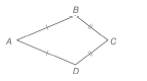Chapter 4.2, Problem 32EElementary Geometry For College St...

7th Edition
Alexander + 2 others
ISBN: 9781337614085

Solutions

Chapter
SectionElementary Geometry For College St...

7th Edition
Alexander + 2 others
ISBN: 9781337614085
Textbook Problem

For Exercises 32 to 35, consider kite A B C D with A B ¯ ≅ A D ¯ and B C ¯ ≅ D C ¯ .For kite A B C D , m ∠ B = 3 x 2 + 2 and m ∠ D = 9 x 4 - 3 .Find x

To determine

To find:

The value of x.

Explanation

Calculation:

Given,

The kite ABCD with

mB=3x2+2 and

mD=9x4-3

Since, a pair of opposite angles are equal in a kite which lie between the sides of unequal lengths of adjacent sides, we have

mB=mD

3x2+2=9x4-3

Add; -9x4 on both sides,

3x2+2+-9x4=9x4-3+-9x4

3x2+-9x4+2=9x4+-9x4+-3

3x2·22+

Still sussing out bartleby?

Check out a sample textbook solution.

See a sample solution

The Solution to Your Study Problems

Bartleby provides explanations to thousands of textbook problems written by our experts, many with advanced degrees!

Get Started

In Exercises 4756, solve the given equation for the indicated variable. 27=32x1

Finite Mathematics and Applied Calculus (MindTap Course List)

Fill in each blank: 3tbs=tsp

Elementary Technical Mathematics

Intervals Express each set in interval notation. 60. (a) (b)

Precalculus: Mathematics for Calculus (Standalone Book)

Express the function in the form f g h. 51. S(t) = sin2(cos t)

Single Variable Calculus: Early Transcendentals, Volume I

Write each expression in terms of i. 18

Trigonometry (MindTap Course List)

For 0

Study Guide for Stewart's Multivariable Calculus, 8th

limx(lnx)1/x= a) 0 b) 1 c) e d)

Study Guide for Stewart's Single Variable Calculus: Early Transcendentals, 8th